Ex 4.1

Chapter 4 Class 11 Mathematical Induction
Serial order wise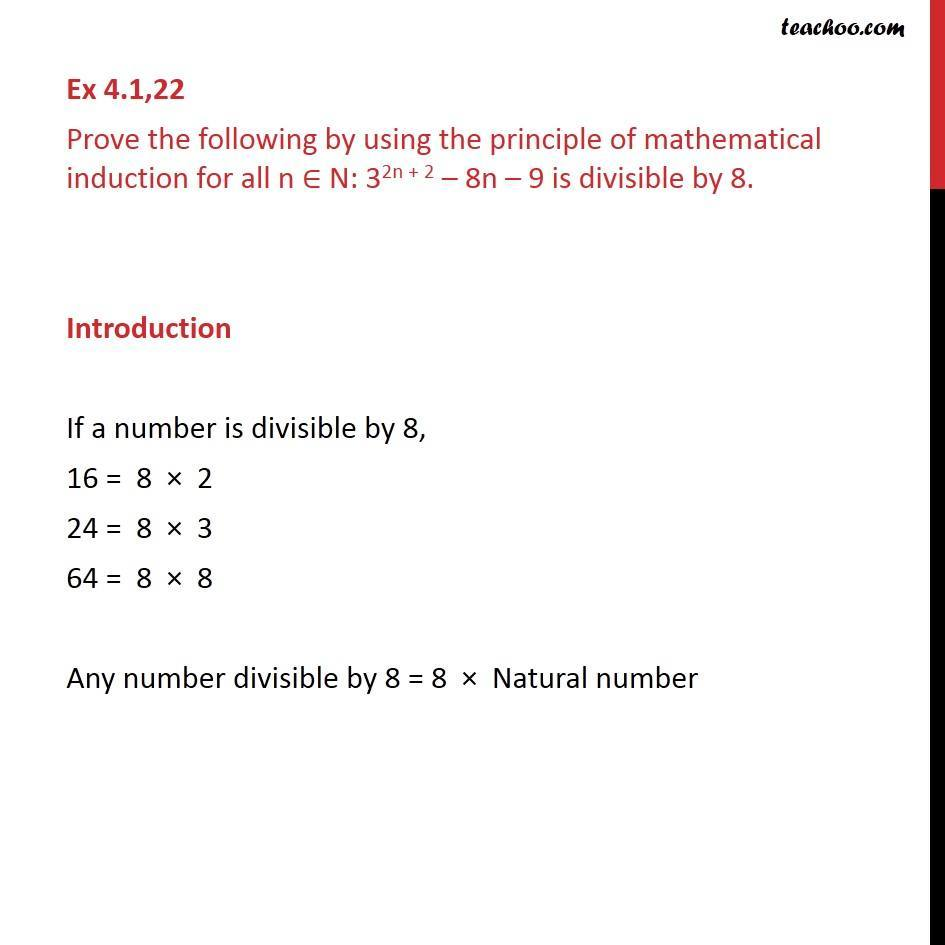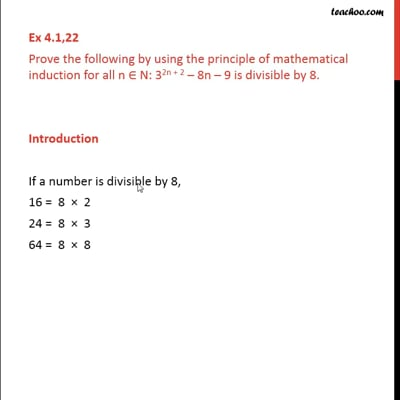This video is only available for Teachoo black users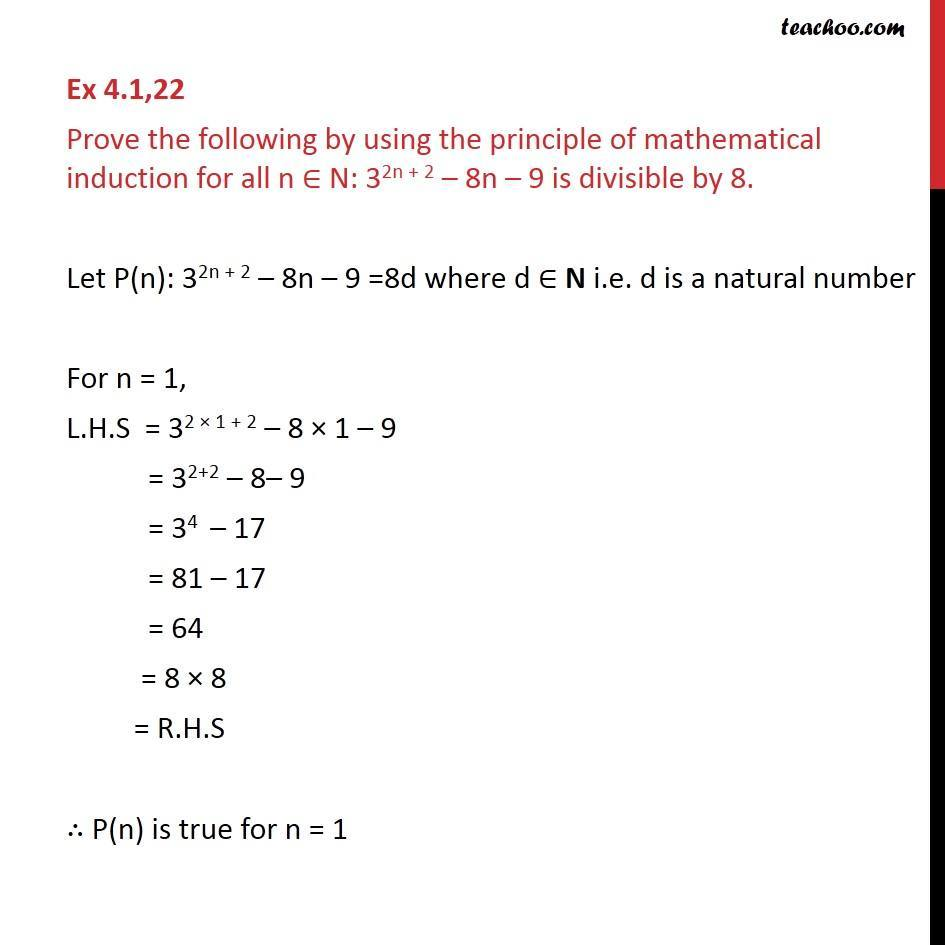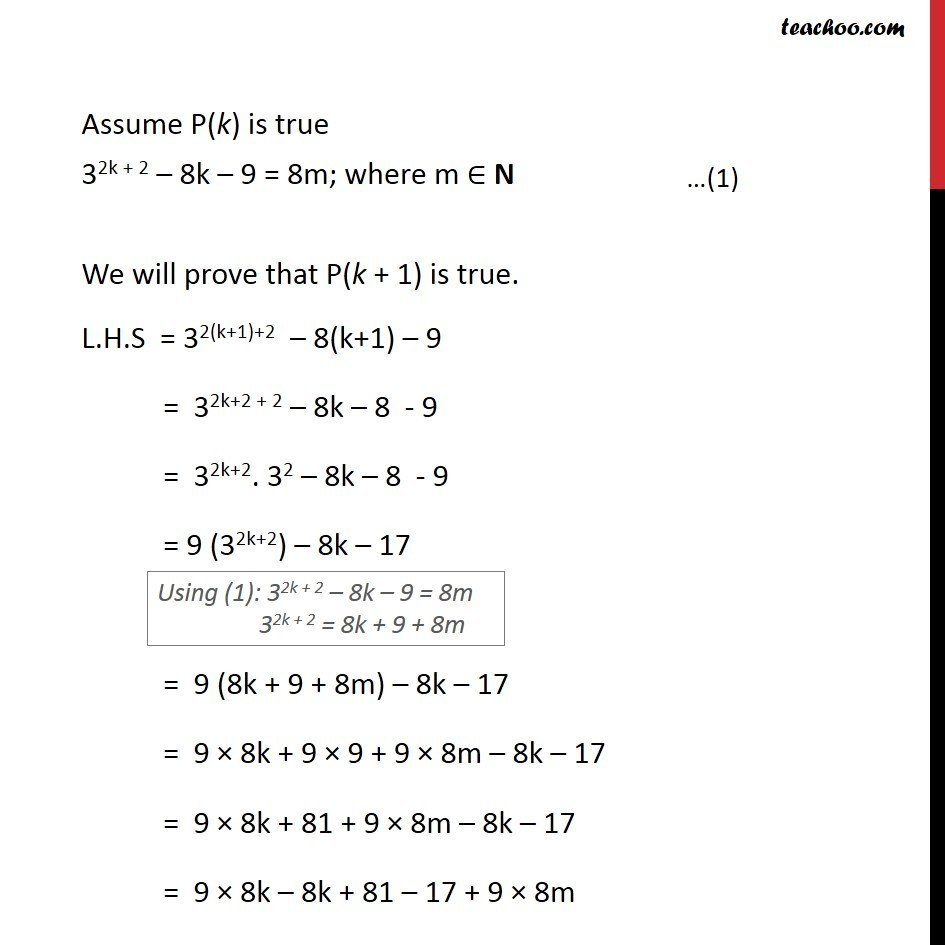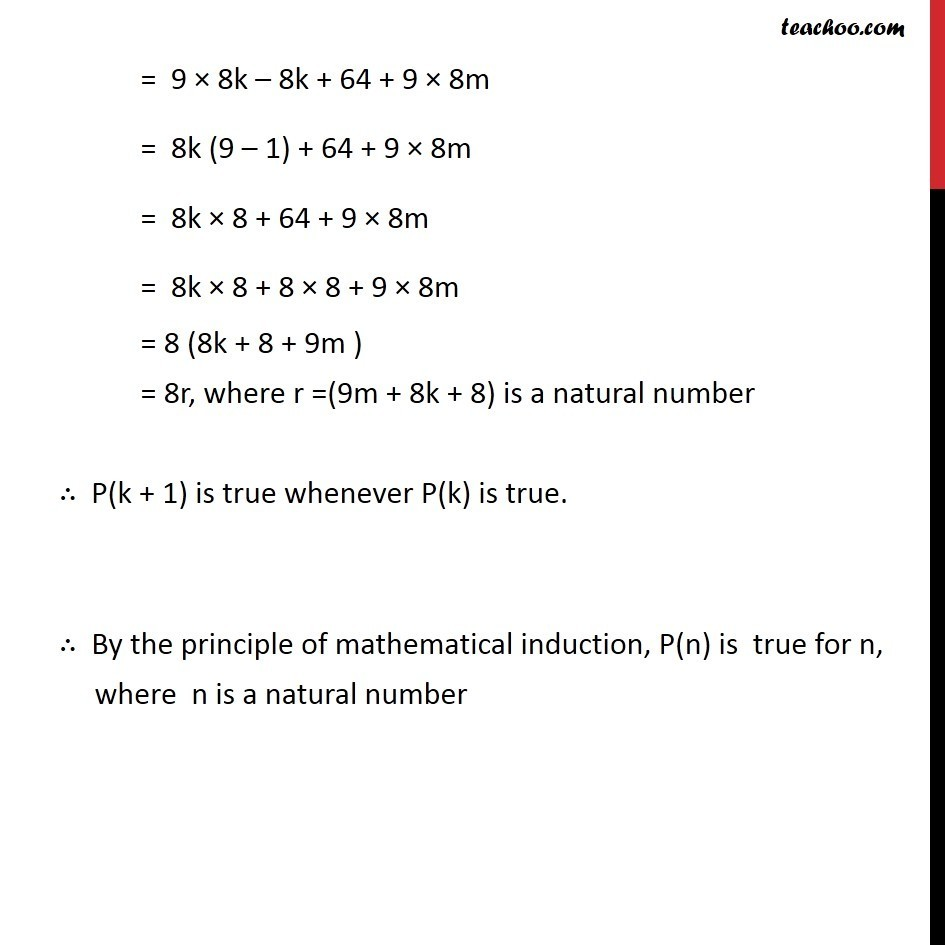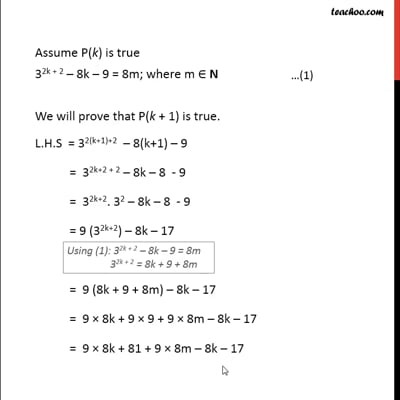This video is only available for Teachoo black users

Solve all your doubts with Teachoo Black (new monthly pack available now!)

### Transcript

Ex 4.1,22 Prove the following by using the principle of mathematical induction for all n N: 32n + 2 8n 9 is divisible by 8. Introduction If a number is divisible by 8, 16 = 8 2 24 = 8 3 64 = 8 8 Any number divisible by 8 = 8 Natural number Ex 4.1,22 Prove the following by using the principle of mathematical induction for all n N: 32n + 2 8n 9 is divisible by 8. Let P(n): 32n + 2 8n 9 =8d where d N i.e. d is a natural number For n = 1, L.H.S = 32 1 + 2 8 1 9 = 32+2 8 9 = 34 17 = 81 17 = 64 = 8 8 = R.H.S P(n) is true for n = 1 Assume P(k) is true 32k + 2 8k 9 = 8m; where m N We will prove that P(k + 1) is true. L.H.S = 32(k+1)+2 8(k+1) 9 = 32k+2 + 2 8k 8 - 9 = 32k+2. 32 8k 8 - 9 = 9 (32k+2) 8k 17 = 9 (8k + 9 + 8m) 8k 17 = 9 8k + 9 9 + 9 8m 8k 17 = 9 8k + 81 + 9 8m 8k 17 = 9 8k 8k + 81 17 + 9 8m = 9 8k 8k + 64 + 9 8m = 8k (9 1) + 64 + 9 8m = 8k 8 + 64 + 9 8m = 8k 8 + 8 8 + 9 8m = 8 (8k + 8 + 9m ) = 8r, where r =(9m + 8k + 8) is a natural number P(k + 1) is true whenever P(k) is true. By the principle of mathematical induction, P(n) is true for n, where n is a natural number